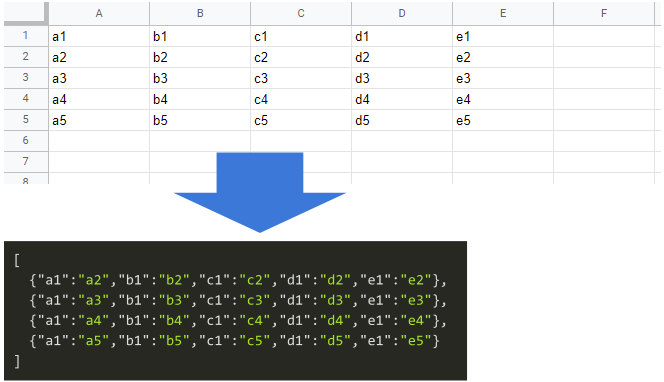GistsThis is a sample script for converting the values of Google Spreadsheet to an object using Google Apps Script.

## Sample script

function myFunction() {
const sheetName = "Sheet1";
const res = rows.map((r) => headers.reduce((o, h, j) => Object.assign(o, { [h]: r[j] }), {}));
console.log(res);
}

• When this script is run, the above sample image can be retrieved.

• In this sample script, the 1st row of the sheet is used as the header row.

• const res = rows.map((r) => headers.reduce((o, h, j) => Object.assign(o, { [h]: r[j] }), {})); can be also replaced with const res = rows.map((r) => headers.reduce((o, h, j) => (o[h] = r[j], o), {}));.

• Also, const res = rows.map((r) => headers.reduce((o, h, j) => Object.assign(o, { [h]: r[j] }), {})); can be replaced with const res = rows.map((r) => Object.fromEntries(headers.map((h, j) => [h, r[j]])));.

When you want to convert a 2-dimensional array to an object array and vice versa, you can also use the following script.

function myFunction() {
const sheetName = "Sheet1";

// Convert 2-dimentional array to object array.
const obj = rows.map((r) => headers.reduce((o, h, j) => (o[h] = r[j], o), {}));
console.log(obj);

// Convert object aray to 2-dimensional array.
console.log(ar);
}


For example, for the above sample Spreadsheet, when the same header titles are included, the sample script is as follows.

function myFunction() {
const sheetName = "Sheet1";

When this script is run, each value is put into an array like [{"a1":["a2"],"b1":["b2a","b2b","b2c"],"c1":["c2"],"d1":["d2"],"e1":["e2"]},,,,].